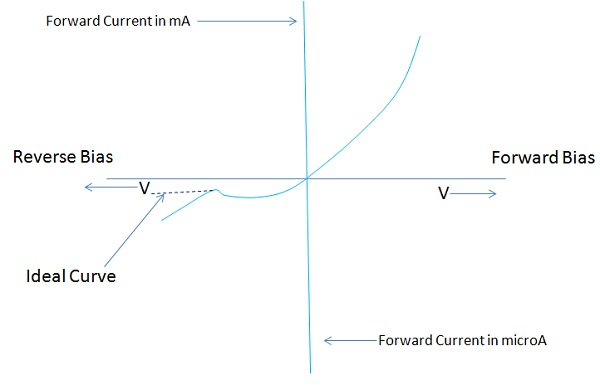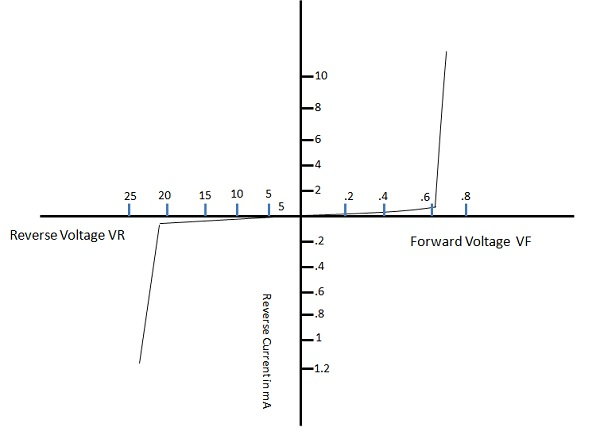# Diode Characteristics

There are diverse current scales for forward bias and reverse bias operations. The forward portion of the curve indicates that the diode conducts simply when the P-region is made positive and the N-region negative.

The diode conducts almost no current in the high resistance direction, i.e. when the Pregion is made negative and the N-region is made positive. Now the holes and electrons are drained away from the junction, causing the barrier potential to increase. This condition is indicated by the reverse current portion of the curve.

The dotted section of the curve indicates the ideal curve, which would result if it were not for avalanche breakdown. The following figure shows the static characteristic of a junction diode.## DIODE IV Characteristics

The forward and reverse current voltage (IV) characteristics of a diode are generally compared on a single characteristic curve. The figure depicted under the section Forward Characteristic shows that Forward Voltage and Reverse Voltage are usually plotted on the horizontal line of the graph.

Forward and reverse current values are shown on the vertical axis of the graph. Forward Voltage represented to the right and Reverse Voltage to the left. The point of beginning or zero value is at the center of the graph. Forward Current lengthens above the horizontal axis with Reverse Current extending downward.

The combined Forward Voltage and Forward Current values are located in the upper right part of the graph and Reverse Voltage and Reverse Current in the lower left corner. Different scales are normally used to display forward and reverse values.

## Forward Characteristic

When a diode is forward biased it conducts current (IF) in forward direction. The value of IF is directly dependent on the amount of forward voltage. The relationship of forward voltage and forward current is called the ampere-volt, or IV characteristic of a diode. A typical diode forward IV characteristic is shown in the following figure.Following are the observations −

• Forward Voltage is measured across the diode and Forward Current is a measure of current through the diode.

• When the forward voltage across the diode equals 0V, forward current (IF) equals 0 mA.

• When the value starts from the starting point (0) of the graph, if VF is progressively increased in 0.1-V steps, IF begins to rise.

• When the value of VF is large enough to overcome the barrier potential of the P-N junction, a considerable increase in IF occurs. The point at which this occurs is often called the knee voltage VK. For germanium diodes, VK is approximately 0.3 V, and 0.7 V for silicon.

• If the value of IF increases much beyond VK, the forward current becomes quite large.

This operation causes excessive heat to develop across the junction and can destroy a diode. To avoid this situation, a protective resistor is connected in series with the diode. This resistor limits the forward current to its maximum rated value. Normally, a currentlimiting resistor is used when diodes are operated in the forward direction.

## Reverse Characteristic

When a diode is reverse biased, it conducts Reverse current that is usually quite small. A typical diode reverse IV characteristic is shown in the above figure.

The vertical reverse current line in this graph has current values expressed in microamperes. The amount of minority current carriers that take part in conduction of reverse current is quite small. In general, this means that reverse current remains constant over a large part of reverse voltage. When the reverse voltage of a diode is increased from the start, there is a very slight change in the reverse current. At the breakdown voltage (VBR) point, current increases very rapidly. The voltage across the diode remains reasonably constant at this time.

This constant-voltage characteristic leads to a number of applications of diode under reverse bias condition. The processes which are responsible for current conduction in a reverse-biased diode are called as Avalanche breakdown and Zener breakdown.

## Diode Specifications

Like any other selection, selection of a diode for a specific application must be considered. Manufacturer generally provides this type of information. Specifications like maximum voltage and current ratings, usual operating conditions, mechanical facts, lead identification, mounting procedures, etc.

Following are some of the important specifications −

• Maximum forward current (IFM) − The absolute maximum repetitive forward current that can pass through a diode.

• Maximum reverse voltage (VRM) − The absolute maximum or peak reverse bias voltage that can be applied to a diode.

• Reverse breakdown voltage (VBR) − The minimum steady-state reverse voltage at which breakdown will occur.

• Maximum forward surge current (IFM-surge) − The maximum current that can be tolerated for a short interval of time. This current value is much greater than IFM.

• Maximum reverse current (IR) − The absolute maximum reverse current that can be tolerated at device operating temperature.

• Forward voltage (VF) − Maximum forward voltage drop for a given forward current at device operating temperature.

• Power dissipation (PD) − The maximum power that the device can safely absorb on a continuous basis in free air at 25° C.

• Reverse recovery time (Trr) − The maximum time that it takes the device to switch from on to off stat.

### Important Terms

• Breakdown Voltage − It is the minimum reverse bias voltage at which PN junction breaks down with sudden rise in reverse current.

• Knee Voltage − It is the forward voltage at which the current through the junction starts to increase rapidly.

• Peak Inverse Voltage − It is the maximum reverse voltage that can be applied to the PN junction, without damaging it.

• Maximum Forward Rating − It is the highest instantaneous forward current that a PN junction can pass, without damaging it.

• Maximum Power Rating − It is the maximum power that can be dissipated from the junction, without damaging the junction.